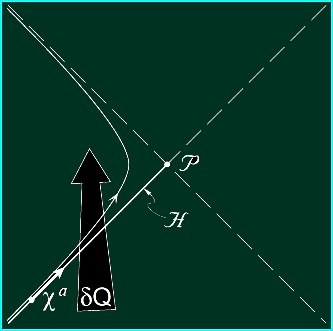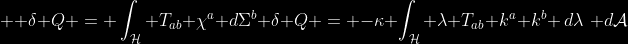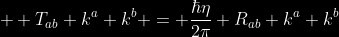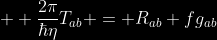## Sunday, March 08, 2009 ...//### Einstein's equations as equations of state

Marco Frasca has pointed out a new paper by Brustein and Hadad that generalizes a cute paper by

Ted Jacobson (1995)
to the case of gravitating Lagrangians with arbitrary couplings of matter, metric, and curvature tensors. Because this new advance is technical and incremental, it may be more useful to discuss Jacobson's original paper instead.

Reviewing Jacobson's finding

The goal was to derive Einstein's equations, i.e. equations relating the Ricci tensor and the energy-momentum tensor, from the thermodynamic equation δQ=T dS. Is that possible? You bet. Normally, we are only used to relate the area and the entropy (or gravitational acceleration with temperature) for static and other simple situations. But Einstein's equations can actually be derived from thermodynamics in the most general time-dependent situation, too.Consider a point P in a curved D-dimensional spacetime, and find the null boundary of its causal past. Treat this (D-1)-dimensional light-like hypersurface as the boundary of a system that is taken to be outside the light cone. Divide this hypersurface into small elementary areas.

Now ask how much energy δQ is crossing the elementary area if you move the hypersurface by a small amount, as measured by the affine parameter δλ. The infinitesimal energy will be proportional to the elementary area as well as δλ and the stress-energy tensor, as well as two copies of the light-like direction defining the null slice and the acceleration of the Killing orbit κ.On the other hand, one wants to calculate T dS. The change of the entropy is related to the area and the temperature is the temperature seen by an Unruh observer. If you combine all the bits and pieces, the universal validity of δQ=T dS requires that for every null D-dimensional vector k, defining the direction of the null hypersurface at a given point, the following relation must hold:It follows thatThe last term proportional to the metric tensor is allowed because it gives you zero when contracted with two copies of any null vector k. The function f is determined to be f = -R/2 + Λ where the cosmological constant Λ remains as undetermined as ever and where the Ricci scalar term has to be the main part of f because of the local conservation of the stress energy tensor (a vanishing covariant divergence).

The multiplicative coefficient η is determined to be 1 / (4 ℏ G), by additional arguments.

Jacobson's derivation has been generalized in various ways and the newest paper extends it to all theories with Lagrangians that are arbitrary functions of matter fields, the metric tensor, the Riemann tensor, and their contractions (I suppose that arbitrary derivatives are not allowed at this moment).

Does gravity have to be quantized?

All this stuff is very nice. But there's some nonsense in Jacobson's original paper, too. The last sentence of the abstract, which is repeated at various places in the paper, states that:
This perspective suggests that it may be no more appropriate to canonically quantize Einstein's equations than it would be to quantize the wave equation for sound in air.
You should ask: WTF? :-) Make no mistake about it: Jacobson wants to argue that gravity shouldn't be quantized at all. Does it make sense? Does it follow from his derivation of Einstein's equations? Is his comment correct at least in the case of the sound?

The answers to these questions are No, No, No. First, quantum mechanics cannot co-exist with classical deterministic physics. Once certain observables fail to be given by deterministic laws, and follow the quantum probabilistic prescriptions instead, the same thing must hold for all other observables that can be influenced by the quantum observables from the beginning of this sentence.

Once you add hats above some quantities, similar hats must appear above all other quantities that are related to them by various equations. So Jacobson is clearly wrong in the case of the sound, too. If you write the wave equation for the pressure or the density of the air, and you define the density of the air to be proportional to the number of molecules N in a squared millimeter of space, it is very clear that the pressure or N also become quantum observables with hats once you switch to a quantum description.

What is true in the case of the air is that there is a lot of other degrees of freedom besides these coarse-grained numbers N in relatively large regions of space. So it would be foolish to think that if you quantize the pressure, you get a full description of the air. This situation differs from the situation of solids at low temperatures where phonons are literally quantized units of sound that may exist above a unique ground state.

Is the ground state unique in the case of quantum gravity? Well, the Minkowski vacuum surely is unique. If it were not unique, it would carry some entropy that would have to be distributed as an entropy density. And the density would have to be the time-like component of a D-dimensional vector, a current, and its nonzero value would break the Lorentz invariance, anyway. So the entropy has to be zero.

It follows that the empty spacetime must be unique and must be analogous to a very cool solid whose excitations are fully described by quantized phonons, i.e. quantum units of sound. The second quantization is a perfectly valid method to construct the Hilbert space and study the quantum dynamics.

On the other hand, when you describe a part of the spacetime only, e.g. if you remove the past light cone of the point P we discussed previously, you implicitly allow the interior of the light cone to contain anything you want. There are many microstates of this type and the corresponding entropy is high. In that case, the gravitating system is analogous to the air that has many more degrees of freedom besides the pressure averaged over the squared millimeter.

A theory of quantum gravity must account for this huge entropy that can be carried by event horizons and similar regions of space. But Jacobson's argument says nothing about the microscopic origin of this entropy. It only tells us that in the thermodynamic limit, i.e. once you compute the averages of the stress-energy tensor and the geometry over large enough regions of space, and if you assume the general area-entropy and acceleration-temperature relationships, Einstein's equations locally follow from the equations of thermodynamics.

Let me emphasize that Jacobson couldn't have derived the equations if he hadn't assumed the equivalence between the area and the entropy - or the acceleration and the temperature. These two (related) relationships know "everything" about the thermodynamic limit of gravitating systems and Jacobson's paper is nothing else than a proof of this statement of mine. It can't tell us anything about quantum gravity before the thermodynamic limit is taken.

Jacobson's argument surely doesn't imply that spacetime is made out of air or a classical material or anything like that, even though Jacobson would clearly like to secretly attach this incorrect conclusion to his otherwise fine argument. He would also like to use his valuable 1995 paper to defend some work of his about the aether and similar crap. ;-)

Despite these tricks, his method is the most complete approach we know that shows that the analogy between general relativity and thermodynamics is more than just an analogy: in the gravitational context, the analogy is actually an identity that holds in every small (but not too small) corner of spacetime.

And that's the memo.

P.S.: The CodeCogs image LaTeX formulae looked pretty nice but I still prefer MathJax now...